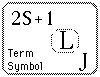# Term Symbols

 The heirarchy of labels for the electrons of multi-electron atoms is configuration, term, level, and state. The term uses the multiplicity 2S + 1, total orbital angular momentum L, and total angular momentum J. It assumes that all the spins combine to produce S, all the orbital angular momenta couple to produce L, and then the spin and orbital terms combine to produce a total angular momentum J. The angular momentum symbol follows the spectroscopic notation scheme. Different terms will in general have different energies, and the order of those energies is usually that given by Hund's Rules, although there are exceptions. The different terms for a given configuration are obtained by forming the different combinations of angular momenta for the electrons outside closed shells, making sure the Pauli Exclusion Principle is obeyed.Index

Atomic Structure Concepts

 HyperPhysics***** Quantum Physics R Nave
Go Back